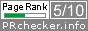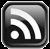# LEARN MS-ACCESS TIPS AND TRICKS

Learn Microsoft Access Advanced Programming Techniques, Tips and Tricks.

### Introduction.

#### The IIF() Function.

The IIF() Function definition is reproduced here from Microsoft Visual Basic for Applicatiions Reference below:

IIf Function Returns one of two parts, depending on the evaluation of an expression.

Syntax: IIf(expr, truepart, falsepart)

The IIf function syntax has these named arguments:

Part Description
expr Required. Expression you want to evaluate.
truepart Required. Value or expression returned if expr is True.
falsepart Required. Value or expression returned if expr is False.

Remarks

IIf always evaluates both truepart and falsepart, even though it returns only one of them. Because of this, you should watch for undesirable side effects. For example, if evaluating falsepart results in a division by zero error, an error occurs even if expr is True.

Example

This example uses the IIf function to evaluate the TestMe parameter of the CheckIt procedure and returns the word "Large" if the amount is greater than 1000; otherwise, it returns the word "Small".

```Function CheckIt(TestMe As Integer)
CheckIt = IIf(TestMe > 1000, "Large", "Small")
End Function ```

Courtesy: Microsoft Access Help

Let us expand the above function to check a series of expressions to return one of the values among them.

```Function CheckIt(TestMe As Integer)
CheckIt = IIF(TestMe > 0 AND TestMe < 256, "Byte",IIF(TestMe > 255 AND TestMe < 32768,"Integer","Large"))
End Function ```

As you can see from the above example that for testing of each expression we have to nest the IIF() function one within another, when two or more expressions are evaluated, and it is absolutely necessary that the parentheses are pared properly.  This is where we face problems while using this function in Query columns or in criteria rows etc.

If we need a valid value to be returned when none of the expressions evaluated to True (in the above example the text "Large") then IIF() is the correct solution, otherwise we have a better function Switch().

### The Switch Function.

The Switch() Function is very simple to use without nesting of expressions like IIF().  Let us rewrite the CheckIt() Function with Switch() function to see how simple it is.

```Function CheckIt(TestMe As Integer)
CheckIt = Switch(TestMe > 0 AND TestMe < 256, "Byte",TestMe > 255 AND TestMe < 32768,"Integer")

End Function ```

The above function does the same work but if none of the expressions evaluated to True then the result returned in CheckIt variable is Null instead of the text "Large".  If you want to check the returned result for Null and replace Null with the text "Large" then rewrite the expression as below:

`CheckIt = NZ(Switch(TestMe > 0 AND TestMe < 256, "Byte",TestMe > 255 AND TestMe < 32768,"Integer"),"Large")`

OR

`x = Switch(TestMe > 0 AND TestMe < 256, "Byte",TestMe > 255 AND TestMe < 32768,"Integer")`

CheckIt = Nz(x,"Large")

If you are using the Switch() Function in a Query column or criteria row then the first example must be used with Switch() enveloped in Nz().

I think it is easier and compact to use Switch() Function when compared with IIF() that needs repeating the function name IIF with balancing of several parenthesis when several expressions are evaluated.

Usage in a sample Query:
```UPDATE Employees SET Employees.Designation = Switch(Employees.Designation IS NULL,'UNKNOWN')
WHERE ((Employees.FirstName IS NOT NULL));```

See the Switch() Function definition given below for details, taken from Microsoft Access Help Document.

Switch Function Evaluates a list of expressions and returns a Variant value or an expression associated with the first expression in the list that is True.

Syntax;

Switch(expr-1, value-1[, expr-2, value-2 … [, expr-n,value-n]])

The Switch function syntax has these parts:

Part Description
expr Required. Variant expression you want to evaluate.
value Required. Value or expression to be returned if the corresponding expression is True.

Remarks:

The Switch function argument list consists of pairs of expressions and values. The expressions are evaluated from left to right, and the value associated with the first expression to evaluate to True is returned. If the parts aren't properly paired, a run-time error occurs. For example, if expr-1 is True, Switch returns value-1. If expr-1 is False, but expr-2 is True, Switch returns value-2, and so on.

Switch returns a Null value if: None of the expressions is True. The first True expression has a corresponding value that is Null. Switch evaluates all of the expressions, even though it returns only one of them. For this reason, you should watch for undesirable side effects. For example, if the evaluation of any expression results in a division by zero error, an error occurs.

Example:

This example uses the Switch function to return the name of a language that matches the name of a city.

```Function MatchUp (CityName As String)

Matchup = Switch(CityName = "London", "English", CityName _
= "Rome", "Italian", CityName = "Paris", "French")
End Function```
Share:

1.how do one can set exact text box width to display text of record for that field.

Could you pls suggest a good book to start learning MSAccess Programming for Beginners (who don't have any background or basic knowledge of programming) or have U written any book.

H Nilesh

2.Answering your first question, if the text box width is one inch then you can display approximately 12 characters (fixed width characters).

Basically there are two types of Fonts: 1) Fixed width fonts 2) Proportional width fonts.
1. Fixed width fonts (e.g. Courier New) takes up equal space for W and I in character mapping. When W I fonts are written next to each other there will be more empty space around the character I.

2. Proportional Fonts (Arial, Times New Roman) takes up only enough area required for the design of each character. W or X characters occupies more space where as I takes up less space.

If you use the text box font size larger than 10 or 12 points then you will be able to display less no of characters. Depending on the field size you can make an approximate calculation for the width of a text box.

On the Reports, on an 80 column (8.5 inch width letter type paper) Paper you can print 80 characters on a line (including spaces i.e. 10 character per inch). When the pitch is set as 12 character per inch then you can print 96 characters on a line. Six lines are printed on one inch height of the paper.

You may buy the book MASTERING ACCESS 2010 (or whatever version 2007 or 2002-2003 of Acces you are using) from any Computer Bookshop. This book is re-published (original publisher Cybex) by BPB Publications, 20 Ansari Road, Darya Ganj, New Delhi-110002. This costs around Rs.400-450.

You will get this book from any good bookshop in major cities.

3.Comments subject to moderation before publishing.

## TranslateSubscribe in a readerYour email address:

Delivered by FeedBurner